More options for analytical torque computation

Last time, we covered the simple airgap model: a cylindrical airgap, with uniform length. We also learned how the stator and rotor currents and magnets were reduced into surface currents, allowing us to focus exclusively on the gap.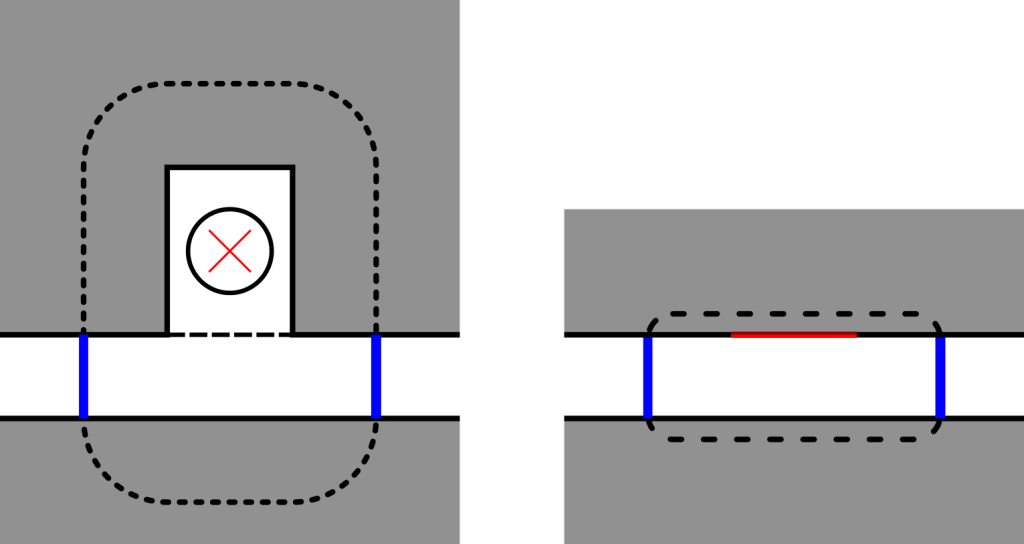Finally, we introduced the concept of the magnetomotive force (mmf)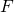. This allowed us to express the airgap (radial) flux density simply as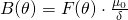,

at the circumferential position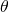.

### Permeance model

However, this simple model suffers from some limitations.

First of all, it doesn’t yet consider the effect of stator slotting – a major contributor to torque ripple and rotor slotting both. Now, the slotting effects could be considered exactly, by modifying the surface currents in a suitable fashion. However, this would make them dependent on the flux density – something we don’t want.

Instead, their effect is approximately included by modifying the airgap length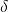. So, our simple model (left, below) is now transformed into this (right):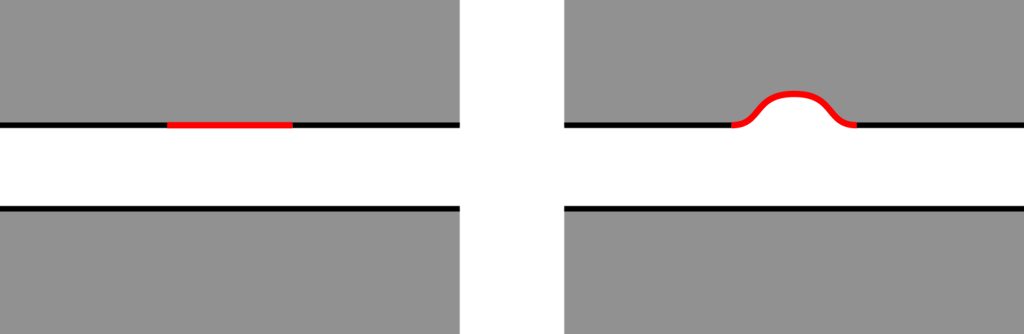Simplest airgap model (left) and a modified model including the effect of slotting (right). The surface current density is highlighted in red.

Nevertheless, the flux density can still be assumed to be approximately proportional to the inverse of the airgap length. Correspondingly, the earlier equation is transformed into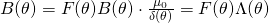Here, the new quantity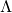is called the (specific) permeance.

Truthfully, permeance is a more general concept than simply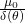. There are several other formulae, of increasing complexity. However, as a first approximation this is just fine.

In practice, the airgap length is written as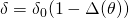where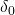is the mean length (enter Carter’s coefficient here), and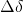is a perturbation. From this, the permeance can be reasonably approximated as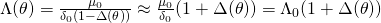.

For a lot more details and info, the book Harmonic Field Effects in Induction Machines is hard to beat. (The link is non-affiliate, but I still recommend the book. If you can find it in a library, better.)

##### Permeance and torque

With this result, the torque calculation can then proceed as before. Often, both mmfs and permeances are written as Fourier expansions.

For example, in a four-pole reluctance motor, the permeance would look like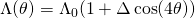After all, there have to be four maxima – one for each pole’s flux to utilize – so hence the figure 4.

The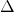term would then interact with the four-pole mmf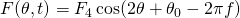,

creating the following rotating flux terms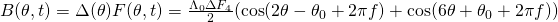.

In other words, flux waves with 4 and 6 poles. The former of these – the 4-pole one – could then interact with the original sinusoidal surface current density, to create torque.

### Where simple airgap models can fail

There is one fundamental assumption with the airgap model as described previously. Indeed, everything outside the gap – meaning rotor and stator – are assumed to be non-segmented iron. Meaning, any flux leaving the airgap is assumed to find an easy return path somewhere else.

Or, if you want to express the same mathematically, the line integral of the field strength outside the airgap is assumed to be zero.

Now, this may not always be the case. A clear example would be a flux-switching machine, illustrated below.

The stator such a machine typically consists of U-shaped iron core segments (grey), sandwiched between permanent magnets (light red) magnetized circumferentially. As permanent magnets have a low permeability (almost equal to air), any flux crossing the magnet requires a large mmf. Hence, it is far easier to “push” flux from one leg of the U-core to the other, compared with core-to-core.

For that reason, creating a simple surface-current model for a FSM is not straightforward. It might be possible, but would require careful analysis to be certain that it’s actually correct.

### Reluctance networks

Luckily, something called reluctance networks rush into the resque. I’ve written about them earlier, but here’s the brief rundown:

Simply, reluctance networks are magnetic equivalent circuits. Instead of resistances, we have reluctances, usually representing the airgap and magnets. Instead of currents flowing in the resistances, we have fluxed. And instead of voltage sources, we have mmf sources: currents and magnets. And (typically), instead of zero-resistance wires we have iron core segments (unless saturation is modelled).

Once the network has been constructed, it can be analysed by well-known circuit analysis, such as the nodal or loop/mesh approaches.

##### Reluctance networks and torque (not really, yet)

However, this does not yet yield the torque straight away. For this purpose, there are several options:

1. Utilize the simple airgap model, with the surface currents fixed such that the field distribution agrees with the reluctance network model.
2. Use the network to calculate inductances, and then use the equivalent circuit approach.
3. Utilize the virtual work principle.
4. Utilize the power balance principle.

Number 1 can be utilized right away, using the methods described so far. However, the two other ones require some extra info – stay tuned for the next post.

Need help with electric motor design or design software? Let's get in touch - satisfaction guaranteed!Options for (analytical) torque computation, part 2

### 2 thoughts on “Options for (analytical) torque computation, part 2”

•Nicola Chiodetto says:

Literally the Heller Hamata “Harmonic Field Effects in Induction Machines” is a holy book.

1.Antti (admin) says:

Indeed!